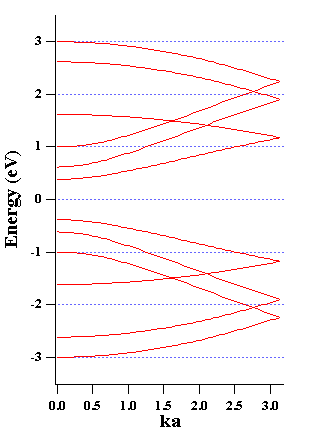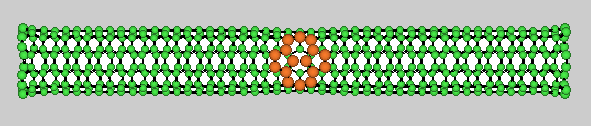# The Nanotube Page

Collaborators: Yuichi Hashi (defects) and Amir A. Farajian (transport)

Electronic properties of carbon nanotubes

All the following calculations were done with a simple tight-binding model with one p orbital per carbon atom. The energies are in units of the hopping integral which is of the order of 2.6 eV.Band structure and DOS of a (4,4) metallic armchair tube.Band structure and DOS of a (5,0) semiconductiong zigzag tube.

Transport in doped nanotubesPicture of a N-P doped (10,0) zigzag nanotube.The conductance of the above tube using a simple approximation to compute its transmission coefficient. The effective voltage W is the sum of the applied voltage and potential difference at the junction (internal voltage).These are the non-linear I-V characteristics of a few doped nanotubes. The calculated conductance is approximate ... but one can notice the strong non-linear effect. (High bias results are incorrect since in this calculation, we have only taken one p orbital per atom, and therefore the DOS goes back to zero at higher energies.) The I-W curve is odd with respect to the EFFECTIVE voltage W, but it is a non-symmetric curve with respect to the applied bias voltage.

Defects (dislocations) in carbon nanotubess1 Stone-Wales transformation5 Stone-Wales transformations10 Stone-Wales transformationsMolecular Dynamics of a streched nanotube at 2000 K after 45 ps.